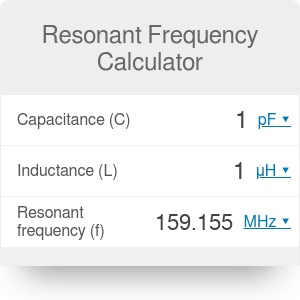Capacitance (C)
pF
Inductance (L)
μH
Resonant frequency (f)
MHz# Resonant Frequency Calculator

By Hanna Pamuła, PhD candidate

If you would like to calculate the resonant frequency of an LC circuit, look no further - this resonant frequency calculator is the tool for you. Enter the inductance and capacitance and in no time at all you'll find the resonant and angular frequency. We also provide some theory as it may be handy - below you'll find out how to calculate resonant frequency as well as a short definition about what the resonant frequency actually is.

If you're interested in electronic circuits, you would probably like to know how to obtain some fraction of input voltage - our voltage divider calculator is a must for that task.

## What is an LC circuit (tank circuit)?

An LC circuit (also called a resonant circuit, tank circuit, or tuned circuit) is an idealized RLC circuit of zero resistance. It contains only an inductor and a capacitor, in a parallel or series configuration:

Tank circuits are commonly used as signal generators and bandpass filters - meaning that they're selecting a signal at a particular frequency from a more complex signal. They are widely applied in electronics - you can find LC circuits in amplifiers, oscillators, tuners, radio transmitters and receivers. LC and RC circuits can be used to filter a signal by blocking certain frequencies.

## What is a resonant frequency?

The resonant frequency is a natural, undamped frequency of a system. If we apply a resonant frequency, then the oscillations become the maximum amplitude, and even relatively small forces can produce large amplitudes. However, if any other frequency is chosen, that signal is dampened. There are many different types of resonances, e.g.

• mechanical and acoustic,
• electrical,
• optical,
• orbital,
• molecular.

For LC circuits, the resonant frequency is determined by the capacitance C and the impedance L.

## How to calculate resonant frequency?

The following formula describes the relationship in an LC circuit:

`f = 1 / (2 * π * √(L * C))`

Where:

• `f` - resonant frequency
• `L` - circuit inductance
• `C` - circuit capacitance

Where does this formula come from? Resonance in the LC circuit appears when the inductive reactance of the inductor becomes equal to the capacitive reactance of the capacitor. So:

• `xL= 2 * π * f * L`
• `xC= 1 / (2 * π * f * C)`

Then, after transforming the equation, we find:

• `xL = xC`
• `2 * π * f * L = 1 / (2 * π * f * C)`
so:
• `f² = 1 / (4 * π² * L* C)`
and finally:
• `f = 1 / (2 * π * √(L * C))`

Also, the angular frequency may be calculated from the following, well-known formula:

`ω = 2 * π * f`

## How to use the resonant frequency calculator

Using our tool is a walk in the park:

1. Enter the capacitor value. For example, our capacitance is equal to 1 μF.
2. Type the inductance. Our inductor in our LC circuit equals 0.18 mH.
3. The resonant frequency calculator did the job! We quickly found out what the resonant frequency is: 11.863 kHz. If you want to check the angular frequency as well, just hit the `Advanced mode` button and the result will appear underneath.

A resonant frequency calculator is a flexible tool, so - as usual - you can type any two variables, and the missing one will be calculated in a flash.

Hanna Pamuła, PhD candidate

## Get the widget!

Resonant Frequency Calculator can be embedded on your website to enrich the content you wrote and make it easier for your visitors to understand your message.

It is free, awesome and will keep people coming back!